Function Repository Resource:

# SumBasis

Find a basis for the sum of a list of subspaces of ℝ^n

Contributed by: Dennis M Schneider
 ResourceFunction["SumBasis"][list1,list2,…] returns a basis for the sum of the subspaces of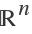spanned by list1,list2,….

## Examples

### Basic Examples (3)

The sum of a 2D subspace and a 3D subspace of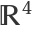:

 In:=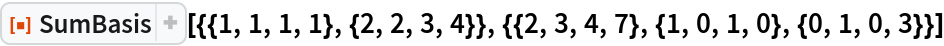Out=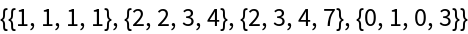The sum of a subspace and a subspace of the subspace:

 In:=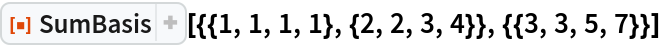Out=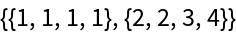A sum of three subspaces:

 In:=Out=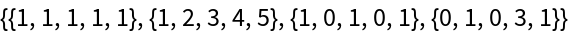## Publisher

Dennis M Schneider

## Version History

• 1.0.0 – 19 September 2019